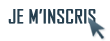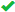Inscrivez-vous gratuitement
pour pouvoir participer, suivre les réponses en temps réel, voter pour les messages, poser vos propres questions et recevoir la newsletter# Discussion: lenteur sur Proc Sql[SQL]

1. ##lenteur sur Proc Sql

Bonjour ,
comment expliquer cette lenteur qui me semble anormale sur l'exécution d'une proc sql
 Code : Sélectionner tout - Visualiser dans une fenêtre à part
```12345678910111213141516171819
proc sql;
create table TDB25 as SELECT  TDB_bul.Code_diplome, TDB_bul.AnSuiv,
sum(eff_app_total17) as eff_app_total17, sum(eff_app_bul_16) as eff_app_bul_16,	sum(eff_app_bul_15) as eff_app_bul_15, sum(eff_app_bul_14) as eff_app_bul_14,
sum(PuEFFECTIF_17) as PuEFFECTIF_17,sum(PrEFFECTIF_17) as PrEFFECTIF_17, sum(EFFECTIF_17Tot) as Scol_17Tot,
sum(PuEFFECTIF_16) as PuEFFECTIF_16,sum(PrEFFECTIF_16) as PrEFFECTIF_16, sum(EFFECTIF_16Tot) as Scol_16Tot,
sum(PuEFFECTIF_15) as PuEFFECTIF_15,sum(PrEFFECTIF_15) as PrEFFECTIF_15, sum(EFFECTIF_15Tot) as Scol_15Tot,
sum(PuEFFECTIF_14) as PuEFFECTIF_14,sum(PrEFFECTIF_14) as PrEFFECTIF_14, sum(EFFECTIF_14Tot) as Scol_14Tot,
sum(PuEFFECTIF_13) as PuEFFECTIF_13,sum(PrEFFECTIF_13) as PrEFFECTIF_13, sum(EFFECTIF_13Tot) as Scol_13Tot,
sum(PuEFFECTIF_12) as PuEFFECTIF_12,sum(PrEFFECTIF_12) as PrEFFECTIF_12, sum(EFFECTIF_12Tot) as Scol_12Tot,

sum(eff_app_gars17) as eff_app_filles17, sum(eff_app_gars17) as eff_app_filles17, sum(eff_app_total17) as eff_app_total17,
/*pas de données en 2016*/
sum(Masc_2015) as Masc_2015 , sum(Fem_2015) as Fem_2015, sum(Masc_2015,Fem_2015) as Eff_App_tot2015,
sum(Masc_2014) as Masc_2014 , sum(Fem_2014) as Fem_2014, sum(Masc_2014,Fem_2014) as Eff_App_tot2014

FROM TDB_bul LEFT JOIN Quater_MF ON TDB_bul.AnSuiv = Quater_MF.Annee_suivie AND TDB_bul.Code_diplome = Quater_MF.Code_diplome
GROUP BY TDB_bul.Code_diplome, TDB_bul.AnSuiv;
run;quit;```
NOTE: The query requires remerging summary statistics back with the original data.
WARNING: La variable eff_app_filles17 existe déjà pour le fichier WORK.TDB25.
WARNING: La variable eff_app_total17 existe déjà pour le fichier WORK.TDB25.
NOTE: Invalid (or missing) arguments to the SUM function have caused the function to return a missing value.
NOTE: Invalid (or missing) arguments to the SUM function have caused the function to return a missing value.
NOTE: Table WORK.TDB25 created, with 2421 rows and 31 columns.

549 run;quit;
NOTE: PROC SQL statements are executed immediately; The RUN statement has no effect.
NOTE: PROCEDURE SQL used (Total process time):
real time 1:53.75
cpu time 1:53.92

Sans la ligne sum(Masc_2014) as Masc_2014 , sum(Fem_2014) as Fem_2014, sum(Masc_2014,Fem_2014) as Eff_App_tot2014
ça donne
NOTE: PROC SQL statements are executed immediately; The RUN statement has no effect.
NOTE: PROCEDURE SQL used (Total process time):
real time 57.54 secondes
cpu time 57.50 secondes

le problème c'est que j'ai encore une dizaine de lignes de code à ajouter (autant de colonnes). Est-ce normal ?
TDB_bul fait 2421 lignes et Quater_MF 1775
NB : je précise que je suis en local , pas en réseaux
Merci de votre réponseRépondre avec citation   0  0

2. ##Bonjour,
Je souhaiterais envoyer en privé à un modérateur ou admin les deux tables utilisées juste pour nous dire la durée de l'exécution. Chez moi ça dure 15 minutes en local, est-ce normal ?
Merci d'avance
 Code : Sélectionner tout - Visualiser dans une fenêtre à part
```123456789101112131415161718192021222324252627proc sql;
create table TDB25 as SELECT  TDB_bul.Code_diplome, TDB_bul.AnSuiv,
sum(eff_app_total17) as eff_app_total17, sum(eff_app_bul_16) as eff_app_bul_16,      sum(eff_app_bul_15) as eff_app_bul_15, sum(eff_app_bul_14) as eff_app_bul_14,
sum(PuEFFECTIF_17) as PuEFFECTIF_17,sum(PrEFFECTIF_17) as PrEFFECTIF_17, sum(EFFECTIF_17Tot) as Scol_17Tot,
sum(PuEFFECTIF_16) as PuEFFECTIF_16,sum(PrEFFECTIF_16) as PrEFFECTIF_16, sum(EFFECTIF_16Tot) as Scol_16Tot,
sum(PuEFFECTIF_15) as PuEFFECTIF_15,sum(PrEFFECTIF_15) as PrEFFECTIF_15, sum(EFFECTIF_15Tot) as Scol_15Tot,
sum(PuEFFECTIF_14) as PuEFFECTIF_14,sum(PrEFFECTIF_14) as PrEFFECTIF_14, sum(EFFECTIF_14Tot) as Scol_14Tot,
sum(PuEFFECTIF_13) as PuEFFECTIF_13,sum(PrEFFECTIF_13) as PrEFFECTIF_13, sum(EFFECTIF_13Tot) as Scol_13Tot,
sum(PuEFFECTIF_12) as PuEFFECTIF_12,sum(PrEFFECTIF_12) as PrEFFECTIF_12, sum(EFFECTIF_12Tot) as Scol_12Tot,
sum(eff_app_gars17) as eff_app_filles17, sum(eff_app_gars17) as eff_app_filles17, sum(eff_app_total17) as eff_app_total17,
sum(Masc_2015) as Masc_2015 , sum(Fem_2015) as Fem_2015, sum(Masc_2015,Fem_2015) as Eff_App_tot2015,
sum(Masc_2014) as Masc_2014 , sum(Fem_2014) as Fem_2014, sum(Masc_2014,Fem_2014) as Eff_App_tot2014,
sum(CAPScoPubl17) as CAPScoPubl17,
sum(CAPScoPubl16) as CAPScoPubl16,
sum(CAPScoPubl15) as CAPScoPubl15,
sum(CAPScoPubl14) as CAPScoPubl14,
sum(PuDIVISIONS_17) as PuDIVISIONS_17, sum(PrDIVISIONS_17) as PrDIVISIONS_17, sum(DIVISIONS_17Tot) as DIVISIONS_17Tot,
sum(PuDIVISIONS_16) as PuDIVISIONS_16, sum(PrDIVISIONS_16) as PrDIVISIONS_16, sum(DIVISIONS_16Tot) as DIVISIONS_16Tot,
sum(PuDIVISIONS_15) as PuDIVISIONS_15, sum(PrDIVISIONS_15) as PrDIVISIONS_15, sum(DIVISIONS_15Tot) as DIVISIONS_15Tot,
sum(PuDIVISIONS_14) as PuDIVISIONS_14, sum(PrDIVISIONS_14) as PrDIVISIONS_14, sum(DIVISIONS_14Tot) as DIVISIONS_14Tot,
sum(PuDIVISIONS_13) as PuDIVISIONS_13, sum(PrDIVISIONS_13) as PrDIVISIONS_13, sum(DIVISIONS_13Tot) as DIVISIONS_13Tot,
sum(PuDIVISIONS_12) as PuDIVISIONS_12, sum(PrDIVISIONS_12) as PrDIVISIONS_12, sum(DIVISIONS_12Tot) as DIVISIONS_12Tot,
Sum(NbrEtabAppr) AS NbrEtabAppr, Sum(NbrEtabScol) AS NbrEtabScol, Sum(NbrEtabScoPub) AS NbrEtabScoPub, Sum(NbrEtabScoPriv) AS NbrEtabScoPriv

FROM TDB_bul LEFT JOIN Quater_MF ON TDB_bul.AnSuiv = Quater_MF.Annee_suivie AND TDB_bul.Code_diplome = Quater_MF.Code_diplome
GROUP BY TDB_bul.Code_diplome, TDB_bul.AnSuiv;
run;quit;```Répondre avec citation   0  0

3. ##J'ai trouvé la solution à une partie de mon problème ici avec la proc summary et dans le cas d'une seule table:
https://communities.sas.com/t5/SAS-P.../451502#M69750

Mais je n'ai pas la réponse à la question de la lenteur avec la proc sql en regroupement avec 2 tables.Répondre avec citation   0  0

4. ##Hello,
As-tu essayé de séparer cette étape en deux et comparer les performances?

Etape 1 : jointure entre les deux tables
Etape 2 : faire une proc summaryRépondre avec citation   0  0

5. ##Envoyé par s_a_mHello,
As-tu essayé de séparer cette étape en deux et comparer les performances?

Etape 1 : jointure entre les deux tables
Etape 2 : faire une proc summary
Désolé, au bout de 2/3 jours, je croyais que personne n'allait répondre , effectivement c'est ce que j'ai fait et ça a marché
https://communities.sas.com/t5/SAS-P...on/td-p/451593
MerciRépondre avec citation   0  0

+ Répondre à la discussion
Cette discussion est résolue.
 Actualités Tutoriels SAS FAQ SAS Livres SAS Ressources Bibliographiques Sources / Macros SAS